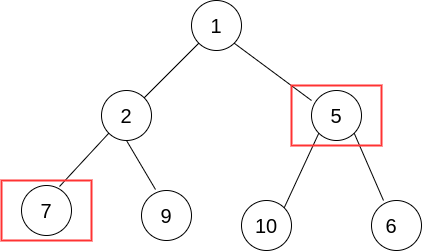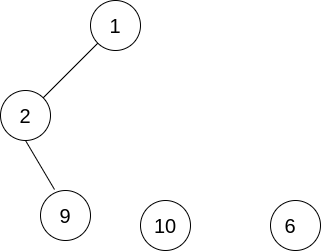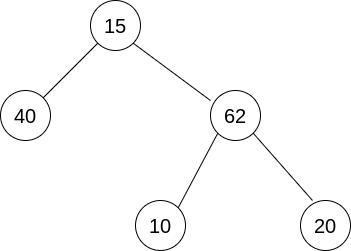# Delete Nodes And Return Forest

Posted: 24 Mar, 2021
Difficulty: Moderate

## PROBLEM STATEMENT

#### Example :#### If the nodes marked in red are deleted we will have the following disjoint trees:#### Note :

``````The order of elements within the lists doesn’t matter.
``````
##### Input Format :
``````The first line of the input contains ‘T’ denoting the number of test cases.

The first line of each test case contains the elements of the tree in the level order form separated by a single space.

The second contains each test case contains an integer ‘K’ denoting the number of elements in the ‘delNodes’ array.

The third line of each test case contains K space-separated integers which are the elements to deleted from the tree.

In the first line values of nodes separated by a single space. In case a node is null, we take -1 in its place.
````````````For example, the level order input for the tree depicted in the above image would be :
15
40 62
-1 -1 10 20
-1 -1 -1 -1
``````

#### Explanation :

``````Level 1 :
The root node of the tree is 15.

Level 2 :
Left child of 15 = 40
Right child of 15 = 62

Level 3 :
Left child of 40 = null (-1)
Right child of 40 = null (-1)
Left child of 62 = 10
Right child of 62 = 20

Level 4 :
Left child of 10 = null (-1)
Right child of 10 = null (-1)
Left child of 20 = null (-1)
Right child of 20 = null (-1)
``````
##### Note :
``````The above format was just to provide clarity on how the input is formed for a given tree.

The sequence will be put together in a single line separated by a single space. Hence, for the above-depicted tree, the input will be given as:
15 40 62 -1 -1 10 20 -1 -1 -1 -1
``````
##### Output Format :
``````For each case just return the list of pointers to the root nodes of disjoint trees,
``````
##### Constraints :
``````1 <= T <= 5
1 <= N <= 3000
1 <= K <= N
0 <= Node Data <= 5000

Time Limit: 1sec
``````Approach 1
• The key idea here is we solve the problem recursively for the left and the right subtree.
• If a node is to be deleted we find the root of the left subtree and if there exists some root in the left subtree ( because it might be possible that all elements on left are deleted) we add it into the final answer, similarly, we do for the right subtree.
• If a node isn’t to be deleted we just traverse left and right subtrees of it.
• For the root of the original tree, we just check if it has to deleted or not.

Algorithm :

• Create a set ‘st’ from the ‘delNodes’ array.
• Check if the value at root is to be deleted, if not then add it into our final answer.
• We call a recursive function DFS( root ). And check if the root is to be deleted.
• If it is to be deleted we recursively call DFS for root->left, and root->right, and then delete root node.
• If root->left is not null ( i.e. it is not deleted) we insert the pointer in our final answer. Similarly, we add root->right if it is not deleted. And return null from the function which tells that the current node is deleted.
• If the current node isn’t deleted we just call root->left and root->right in the DFS function.